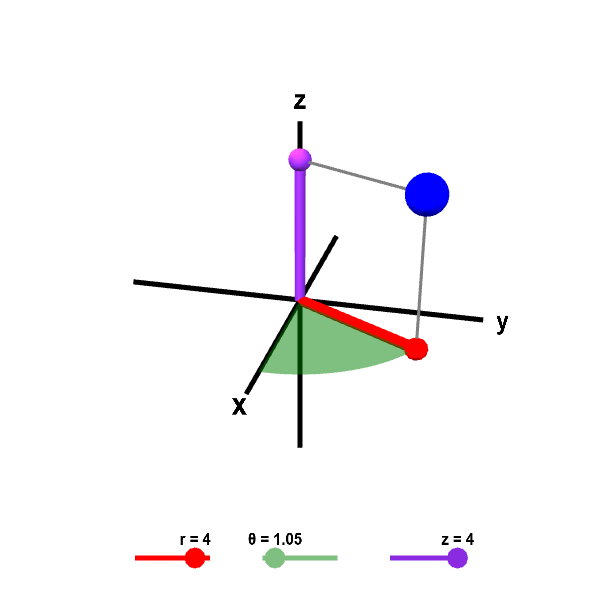# Math Insight

### Cylindrical coordinates

Cylindrical coordinates are a simple extension of the two-dimensional polar coordinates to three dimensions. Recall that the position of a point in the plane can be described using polar coordinates $(r,\theta)$. The polar coordinate $r$ is the distance of the point from the origin. The polar coordinate $\theta$ is the angle between the $x$-axis and the line segment from the origin to the point. Polar coordinates are illustrated in the below figure and described more in another page.

Cylindrical coordinates simply combine the polar coordinates in the $xy$-plane with the usual $z$ coordinate of Cartesian coordinates. To form the cylindrical coordinates of a point $P$, simply project it down to a point $Q$ in the $xy$-plane (see the below figure). Then, take the polar coordinates $(r,\theta)$ of the point $Q$, i.e., $r$ is the distance from the origin to $Q$ and $\theta$ is the angle between the positive $x$-axis and the line segment from the origin to $Q$. The third cylindrical coordinate is the same as the usual $z$-coordinate. It is the signed distance of the point $P$ to the $xy$-plane (being negative is $P$ is below the $xy$-plane). The below figure illustrates the cylindrical coordinates $(r,\theta,z)$ of the point $P$.

You can further explore the properties of the cylindrical coordinates with the follow applet. You can observe how changing the coordinates $(r,\theta,z)$ changes the position of the point $P$. Just as with polar coordinates, we usually limit $0 \le \theta < 2\pi$ and $r \ge 0$ to descrease the non-uniqueness of cylindrical coordinates. However, when $r=0$, there is a non-uniqueness since the point $P$ is on the $z$ axis when $r=0$, independent of the value of $\theta$.Cylindrical coordinates. Given the values for cylindrical coordinates $r$, $\theta$, and $z$, the large blue point shows the corresponding position in Cartesian coordinates. You can change $r$, $\theta$, and $z$ using the sliders or by dragging the other points shown. The red point is the projection of the large blue point in the $xy$-plane. This projection gives perspective and helps in visualizing $\theta$ and $r$. The coordinate $r$ is the length of the red line segment from the origin to the red point. The coordinate $\theta$ is the angle the red line segment makes with the positive $x$-axis; it is the angle of the green portion of the portion of the disk in the $xy$-plane. The coordinate $z$ is the same as the $z$-coordinate of Cartesian coordinates; it is the height of the purple point on the $z$-axis.